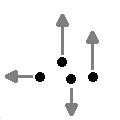CGAL 4.7 - Kinetic Framework
Kinetic Framework ReferenceDaniel Russel
Kinetic data structures allow combinatorial geometric structures to be maintained as the primitives move. The package provides a framework to ease implementing and debugging kinetic data structures. The package supports exact or inexact operations on primitives which move along polynomial trajectories.

Introduced in: CGAL 3.2
Depends on: Two dimensional visualization depends on Qt 3, three dimensional visualization depends on Coin3D.
BibTeX: cgal:r-kdsf-15b
License: LGPL

## Definition

Kinetic data structures are a way of adding motion to classical geometric data structures. CGAL provides a number of classes to aid implementation of kinetic data structures.

There are three levels at which the user can interact with the package. The user can use an existing kinetic data structure, write a new kinetic data structure, or replace parts of the framework. The first level is covered in this Chapter.

## Main Support Classes and Concepts

Here we list the main classes and concepts provided by the framework to support implementing kinetic data structures

• Kinetic::ActiveObjectsTable
• CGAL::Kinetic::Active_objects_vector<MovingObject>
• CGAL::Kinetic::Cartesian<FunctionKernel>
• Kinetic::FunctionKernel
• Kinetic::InstantaneousKernel
• CGAL::Kinetic::Default_instantaneous_kernel<ActiveObjectsTable, StaticKernel>
• Kinetic::Kernel Kinetic::SimulationTraits
• Kinetic::Simulator
• CGAL::Kinetic::Default_simulator<FunctionKernel, EventQueue>

## Other Concepts

• Kinetic::Key
• Kinetic::Certificate
• Kinetic::CertificateGenerator
• Kinetic::EventQueue Kinetic::FunctionKernel::ConstructFunction
• Kinetic::FunctionKernel::Function
• Kinetic::RootStack
• Kinetic::Simulator::Event
• Kinetic::Simulator::Time

## Other Classes

• CGAL::Listener<Interface>
• CGAL::Multi_listener<Interface>
• CGAL::Ref_counted<T>
• CGAL::Kinetic::Active_objects_listener_helper<ActiveObjectsTable, KDS>
• CGAL::Kinetic::Erase_event<ActiveObjectsTable>
• CGAL::Kinetic::Insert_event<ActiveObjectsTable>
• CGAL::Kinetic::Qt_moving_points_2<Traits, QtWidget_2>
• CGAL::Kinetic::Qt_triangulation_2<KineticTriangulation_2, QtWidget_2, QtMovingPoints_2>
• CGAL::Kinetic::Qt_widget_2<Simulator>
• CGAL::Kinetic::Regular_triangulation_instantaneous_kernel<ActiveObjectsTable, StaticKernel>
• CGAL::Kinetic::Simulator_kds_listener<Listener, KDS>
• CGAL::Kinetic::Simulator_objects_listener<Simulator_listener, KDS>

## Example

The simulation traits class is simply there for convenience in order to bundle a set of related typedefs and create a few objects. As a resulting, creating your own requires little though, and just copying and changing a few lines. An example is below which sets up to use the CORE Sturm sequences to solve polynomials rather than our own (faster) solvers. It can be found in examples/Kinetic_framework/defining_a_simulation_traits.cpp.

#include <CGAL/Polynomial/Sturm_root_stack_traits.h>
#include <CGAL/Polynomial/Sturm_root_stack.h>
#include <CGAL/Kinetic/Active_objects_vector.h>
#include <CGAL/Kinetic/Default_instantaneous_kernel.h>
#include <CGAL/Kinetic/Cartesian.h>
#include <CGAL/Kinetic/Handle_degeneracy_function_kernel.h>
#include <CGAL/Kinetic/Default_simulator.h>
#include <CGAL/Kinetic/Two_list_pointer_event_queue.h>
#include <CGAL/Exact_predicates_exact_constructions_kernel.h>
using namespace CGAL::Kinetic;
struct My_simulation_traits {
typedef My_simulation_traits This;
//typedef CGAL::Regular_triangulation_euclidean_traits_3<Static_kernel_base> Static_kernel;
typedef CGAL::POLYNOMIAL::Polynomial<Static_kernel::FT> Function;
typedef CGAL::POLYNOMIAL::Sturm_root_stack_traits<Function> Root_stack_traits;
typedef CGAL::POLYNOMIAL::Sturm_root_stack<Root_stack_traits> Root_stack;
typedef CGAL::POLYNOMIAL::Kernel<Function, Root_stack> Function_kernel;
typedef CGAL::Kinetic::Handle_degeneracy_function_kernel<Function_kernel, false> Simulator_function_kernel_base;
struct Simulator_function_kernel: public Simulator_function_kernel_base{};
typedef Cartesian<Simulator_function_kernel> Kinetic_kernel;
typedef Two_list_pointer_event_queue<Function_kernel> Event_queue;
typedef Active_objects_vector<Kinetic_kernel::Point_1> Active_points_1_table;
typedef Active_objects_vector<Kinetic_kernel::Point_2> Active_points_2_table;
typedef Active_objects_vector<Kinetic_kernel::Point_3> Active_points_3_table;
// typedef Active_objects_vector<Kinetic_kernel::Weighted_point_3> Active_weighted_points_3_table;
typedef Default_instantaneous_kernel<This> Instantaneous_kernel;
Active_points_1_table* active_points_1_table_handle() const { return ap1_.get();}
Active_points_2_table* active_points_2_table_handle() const {return ap2_.get();}
Active_points_3_table* active_points_3_table_handle() const {return ap3_.get();}
//Active_weighted_points_3_table* active_weighted_points_3_table_handle() const {return awp3_.get();}
Simulator* simulator_handle() const { return sim_.get();}
const Static_kernel& static_kernel_object() const {return k_;}
const Kinetic_kernel& kinetic_kernel_object() const {return kk_;}
Instantaneous_kernel instantaneous_kernel_object() const {
return Instantaneous_kernel(*this);
}
My_simulation_traits(const Simulator::Time &lb,
const Simulator::Time &ub): sim_(new Simulator(lb, ub)),
ap1_(new Active_points_1_table()),
ap2_(new Active_points_2_table()),
ap3_(new Active_points_3_table())
//awp3_(new Active_weighted_points_3_table())
{}
bool is_exact() const {
return true;
}
protected:
Simulator::Handle sim_;
Active_points_1_table::Handle ap1_;
Active_points_2_table::Handle ap2_;
Active_points_3_table::Handle ap3_;
//Active_weighted_points_3_table::Handle awp3_;
Static_kernel k_;
Kinetic_kernel kk_;
Function_kernel fk_;
};

## Modules

Main Concepts
Here we list the main concepts provided by the framework to support implementing kinetic data structures.

Main Classes
Here we list the main classes provided by the framework to support implementing kinetic data structures.

Other Classes

Other Concepts